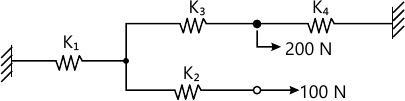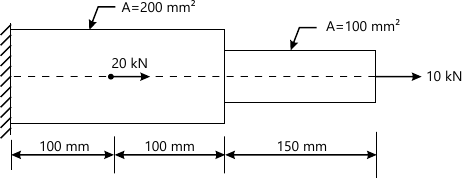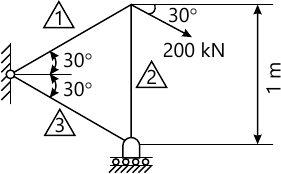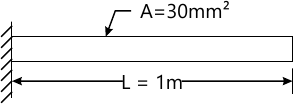MORE IN Finite Element Analysis
MU Mechanical Engineering (Semester 8)
Finite Element Analysis
May 2014
Total marks: --
Total time: --
INSTRUCTIONS
(1) Assume appropriate data and state your reasons
(2) Marks are given to the right of every question
(3) Draw neat diagrams wherever necessary

1(a) What is the difference between shape function and interpolation function?Derive the shape function for cubic bar element in local co-ordinates and sketch the same along the length of the element.
10 M
1(b) A quadrilateral element is defined by the co-ordinates (1,4),(4,2),(5,6) and (2,7).The temperature at the nodes are 20°C,30°C,40°C, and 25°C respectively.Determine the temperature at apoint P(3,4)
10 M

2(a) The following differential equation arises in connection neat transfer through a rectangular fig
$-KA \dfrac{d^{2}T}{dx^{2}} +hp(T-T_{infty})=0; 0 \leq x \leq 1 BCS: T(0) =T(0)$
$KA \dfrac{dT}{dx}+hA(T-T_{infty})]_{x=L}=0$
Develop an element matrix equation for the same linear element.
After getting element matrix equation use following data to find nodal temperature,K=385 W/m.k, h =25 W/m2-k to =100°C T=20°C,L=100mm,b=5mm and t=1mm
Take 4 elements (linear)
15 M
2(b) Explain different types of nodes with suitable examples.
5 M

3(a) Determine the displacement at the nodes principle of minimum potential energy and reaction at the supports
Use; K1=100N/mm, K3=150N/mm and K4=200 N/mm.8 M
3(b) Analyse completely the problem given below; Assume,E =200 Gpa8 M
3(c) What do you mean by pre-processing and post-processing related to FEA software?
4 M

4(a) Analyse the following truss for displacements reaction and stresses
For each element
E=100 Gpa
A=50cm214 M
4(b) Explain different types of boundary condition with suitable examples.
6 M

5(a) Derive shape function for nine noded quadrilateral element in natural co-ordinates.
10 M
5(b) Find the natural frequencies of axial vibration for a bar as shown in figure below using one linear element by using consistent and lumped mass matrices.
take E = 2 × 1011 N/m2 , ρ = 7800 kg/m310 M

6(a) Solve the following differential equation by
Galerkin method
Rayleigh-ritz method over entire domain
$\dfrac{d^{2}u}{dx^{2}}-9u=x^{3}; 0\pm x\pm 1 BCS; U(0)=0 and u(1)=2 Compare the answer with exact solution at x=0.25,0.5 and 0.75. 14 M 6(b) Explain subparametric, isoparamertric and superparametric elements. 6 M 7(a) Evaluate for following integral by using 2 × 2 Guass Quadratic rule \[I= \int_{y=4}^{6} \int_{x=2}^{2} (1-x)^{2}(4-2)^{2}dx dy Compare your answer with exact solution:  No.of Sampling Points ϵi Wi 1 0 2 2 \[\pm \dfrac{1}{\sqrt{3}}\$ 1 3 0 \[\pm \sqrt{0.6} 8/9 5/9
10 M
7(b) What are the sources of error in FEM.
5 M
7(c) Compare FEM with classical methods.
5 M

More question papers from Finite Element Analysis